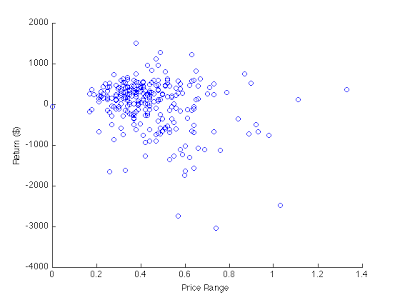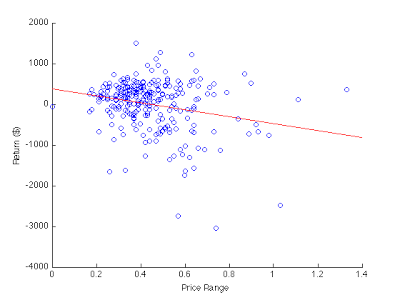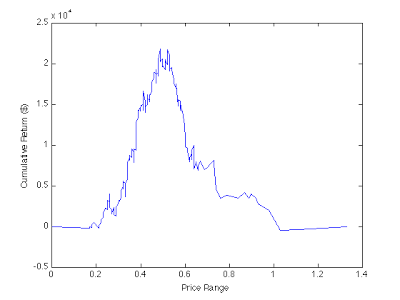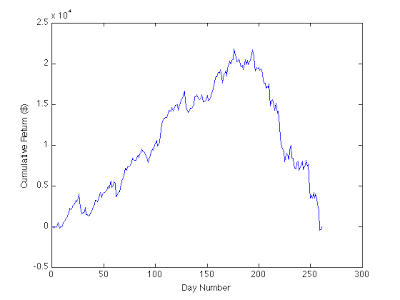# Using Cumulative Sums to Analyze Financial Data

December 21, 2010

The goal of modeling is to be able to describe and predict the behavior of a system. When modeling systems where the general form of the model is unknown, one must usually begin by looking at data to get a sense for the relationship between model performance and potentially explanatory variables. Analyzing financial data is a difficult problem, but one that can often be made easier by finding a useful visualization for the data. This post will use Matlab to demonstrate a handy trick for transforming and visualizing financial time series data that can sometimes quickly reveal hidden features.

The example set of data is a set of returns (normalized to mean zero) for a trading algorithm built to emulate a short straddle on a stock from 4/01/09 to 4/13/10. The columns are date, return in dollars, and a measure of the high-low price range of the instrument being traded. Each row is a single day. Our goal is to try to understand the relationship, if any, between the return of the algorithm and the price range of the underlying instrument.

A scatter plot is a good place to start:

figure(1); scatter(exampleData(:,3),exampleData(:,2)) xlabel('Price Range'); ylabel('Return ($)');Adding the least squares regression line may also be helpful:  A = [exampleData(:,3) ones(length(exampleData),1)]; B = exampleData(:,2); regrCoefficients = lscov(A,B); hold on; plot([0; 1.4], [0 1; 1.4 1]*regrCoefficients,'r'); hold off;A short straddle tends to make money when the price does not move very much, so a higher price range should lead to a higher loss. Thus, a negatively sloped regression line is expected. Other than that it is hard to tell very much from this plot. For a smooth noise-free function, the cumulative or running sum of samples of that function is an approximation of a value proportional to the integral of the function, and so looking at the slope or derivative of this cumulative sum gives an approximation of a value proportional to the original function. This is not very useful for smooth data, but for noisy financial data it can be very revealing: the slope of the cumulative sum of the noisy data is proportional to the instantaneous expectation of the data. Let us sort the data by the price range column, and plot the cumulative sum of the return series: sortedData = sortrows(exampleData,); plot(sortedData(:,3), cumsum(sortedData(:,2))); xlabel('Price Range'); ylabel('Cumulative Return ($)');The above plot shows distinctly that the expectation of our trading algorithm changes rapidly from positive to negative as the price range moves above approximately 0.5. This is close to the zero of our regression line, 0.4461. Note that plotting the data this way, with our sorted price range column as the x-axis, the slope is not the expectation but rather the expectation times the (unnormalized) probability density: a slope may indicate either a large expectation at that price range and a small probability of encountering that price range, or vice versa. So one way to normalize it would be to divide by a probability density function, which could be determined by “bucketing” the data or could be provided exogenously. Another, quicker, way to get a sense for the expectation on a given interval is to plot the same data with even spacing for each point:

plot(1:length(sortedData),cumsum(sortedData(:,2)); xlabel('Day Number'); ylabel('Cumulative Return ($)');The peaks of this and the previous plot correspond to the same point, so by looking at both plots simultaneously we can get a sense for the probability density of the price range distribution and therefore say something about the expectation: while there are fewer negative days than positive days, the magnitude of the expectation on negative days is larger. We can see this because the slope prior to the peak is positive and slope after the peak is negative and larger in magnitude. We can also see that there are only approximately 10 points with a price range higher than 0.75, and these make up approximately$8000 in losses, so our algorithm clearly has a problem dealing with outlier days.

Looking at the slopes of cumulative sums can be a quick, informative way to explore the features of a dataset, as long as one is careful to think about exactly what the slope means in a particular instance. Hopefully you will find these tricks handy in your own analysis of noisy data.